# GEM Geometry File¶

A SIMION geometry file (GEM) defines electrode geometries using constructive solid geometry (CSG) primitives. CSG operations define shapes using unions and intersections of other basic shapes (e.g. a rectangular slab with a cylinder hole cut through it), a bit similar in concept to machining. GEM files are text files and have the file name extension of “.GEM”. SIMION can convert a GEM file to a PA file.

The GEM file appendix in the printed manual is perhaps the first place to start. See also SIMION Example: geometry for examples. “courses\advanced” also has some notes on GEM files. Additional tips are given on this page.

## Learning¶

Where to start learning about GEM files?

Note

This page is abridged from the full SIMION "Supplemental Documentation" (Help file). The following additional sections can be found in the full version of this page accessible via the "Help > Supplemental Documentation" menu in SIMION 8.1.1 or above:
• SIMION 8.1 GEM Syntax Reference

## GEM Extensions in 8.2¶

SIMION 8.2 makes major enhancements to the GEM syntax. Here is a summary of the new features described in more detail below.

• New commands to define shapes easier or with fewer rotations:

• `cylinder3d()` - easily defines a cylinder between two endpoints: `cylinder3d(5,5,0, 5,5,10, 3)`. Use this rather than `cylinder` since it can be simpler and avoids locate rotations.
• `half_space()` - defines a volume below an infinite plane defined by a point and normal vector. `half_space(5,0,0, 1,1,1)`. Use this rather than than `box3d` since it is simpler and avoids rotations.
• `shape()` - defines your own custom shapes from analytical equations or function. Example: `e(1) { shape(function(x,y,z) return x^2 + y^2 > z end)` . Complex surfaces can be defined directly in the GEM file. No need for doing this outside of a GEM file with the `simion.pas pa:fill()` API.
• New transformations:

• `extrude_xy()`, `extrude_yz()` and `extrude_zx()` - extrudes a 2D image drawn in the given plane. For example, `polyline` normally takes an XY plane drawing, which can then be wrapped in a rotation (`locate`) to another plane, but the rotation can be tricky. These commands make it more clear: `extrude_yz() { polyline(0,0, 5,10, 10,0) } ; y,z points` . Even XY extrusions can be specified more simply; for example to extrude from z=3 to z=5: `extrude_xy(3,5) { polyline(0,0, 5,10, 10,0) } ; x,y points` .
• `revolve_xy()`, `revolve_yz()`, `revolve_zx()` - define volumes of rotation in any given plane. These are suggested instead of `rotate_fill`, which only operates in the XY plane without subsequent rotation. This command also defines a shape, so it can be used wherever shapes can be used, such as intersections, which is not possible with `rotate_fill` (except via the workaround of cutting away from a `rotate_fill` using a subsequent `n(0) { }` but that may cut more than the current shape).
• `rotate_x()`, `rotate_y()`, `rotate_z()` - rotates contained objects. They rotate counter-clockwise (CCW) looking down the positive end of the given axis. These are recommended over `locate` commands for clarity. Many times you can omit `rotate_x|y|z` as well when the commands above are used.
• Other command improvements:

• `pa_define()` accepts named parameters. `pa_define {101, 101, dx=0.1}` is equivalent to `pa_define(101,101,1, p, n, e,, 0.1,,,, auto)`. This is recommended. Please note that the `{}` syntax has different defaults than `()`, such as planar non-mirror symmetry and the newer `surface='auto'`. Another example: `pa_define {101, 101, 1, 'planar xy', surface='fractional'}`.
• `pa_define()` can accept mm array sizes rather than grid point sizes. The above example can be rewritten as `pa_define {10*mm, 10*mm, dx=0.1}` . It defines a 10 * 10 mm^2 region. This recommended.
• `e()`: and `n()` can accept a function parameter for defining gradient voltages over electrodes or analytic potentials in space.
• `fill` and `within` can be omitted when not necessary, for shorter GEM code. Use `e(1) { circle(0,0, 50) }` rather than `e(1) { fill { within { circle(0,0, 50) } } }` . See omit fill/within commands.
• `intersect()` is a synonym for `within`. The name `intersect` makes the meaning more clear and is recommended. `e(1) { intersect { box(0,0, 10,10) circle(0,0, 10) } }` .
• `include()` supports improved ways to pass variables to/from included GEM files (see examples below).
• `inf` to represent infinity rather than a large number (e.g. 1e6). `box(-inf,0, inf, 2)`. This is more clear and recommended.
• Simpler syntax:

• `\$(...)` and `#` can be omitted around Lua code. They are no longer necessary:

```local w = 2
e(1) {
for i=1,3 do
box(i*10,0, i*10+w, 10)
end
}
```
• Using a Lua style comment `--` in the file will disable compatibility mode supporting older deprecated GEM syntax such as space-delimited argument lists, `+` before numbers, and unquoted strings. New syntax.

• See New syntax for details on other language changes.

• STL support

• `stl` - include STL files in GEM file. `stl("lens.gem")`. Added in 8.2.0.10.
• Be sure to also utilize SIMION 8.1 features like `surface='auto'` or `surface='fractional'`. See surface=auto option in pa_define [8.1.1.25].

### New syntax¶

The GEM language has been significantly extended to merge GEM syntax, Lua syntax, and prepreprocessing syntax (`# \$()`). Example:

```-- new syntax
pa_define{wx=10, wy=10, wz=10, dx=0.5, surface='fractional'}
local function paraboloid(x0,y0,z0, a, b)
shape(function(x,y,z) return ((x-x0)/a)^2 + ((y-y0)/b)^2 < (z-z0) end)
end
e(1) {
for i=1, 3 do
local y = i*2
box(i*2, 0, i*2+1, y)
end
}
e(2) { paraboloid(5,5,5, 1, 1) }
```

Previously, something like that would require various preprocessing code:

```; old SIMION 8.1 compatible GEM
# local function round(x) return math.floor(x+0.5) end
# local wx, wy, wz = 10, 10, 10
# local dx = 0.5
# local nx = round(wx/dx) + 1
# local ny = round(wy/dx) + 1
# local ny = round(wz/dx) + 1
pa_define(\$(nx), \$(ny), \$(nx), p, n, e,, 0.5, surface=fractional)
# function paraboloid(x0,y0,z0, a)
locate(\$(x0),\$(y0),\$(z0), 1, -90) {
rotate_fill() {
locate(,,, 1, 0,-90) {
within { parabola(0, 0, \$(a^2/4)) }
}
}
}
# end
e(1) {
# for i=1, 3 do
# local y = i*2
fill { within { box(\$(i*2), 0, \$(i*2+1), \$(y)) } }
# end
}
e(2) { \$(paraboloid(5,5,5, 1)) }
```

A more prominent change seen above is that variables and code used in GEM commands need not be escaped anymore with `#` and `\$(...)`, as as these are built into the language now.

Most Lua syntax is now available in GEM, including

• Lua style comments: single line `--` and multiline `--[[...]]`:

```-- this is line comment.
--[[
This is a
multi-line comment.
]]
e(1) { box() }  -- another line comment
```

These are similar to `;` style comments, and the two comments styles must not be mixed in the same file.

• Named parameters can be used with `{` style function calls. This is primary used for the `pa_define()` command:

```pa_define {wx=10, wy=10, dx=0.1, surface='fractional'}
```
• Arbitrary Lua code, outside of commands braces:

```local x = 1
for i=1,10 do
x = x + i
end
print('hello world', x)
e(1) { box(x,x, x+1, x+1) }
```
• `if`, `for` and `local` statements within command brackets:

```e(1) {
box()
for i=1,4 do
local j = i*2
box()
if i % 2 == 0 then
box()
end
box()
circle()
end
polyline(
for i=1,3 do
i, i*2
end
)
}
```

This is convenient for geometric constructions. It is an extension to Lua syntax (normally statements cannot exist inside expressions such as inside braces, but this syntax extension allows it).

Note: Commas may be placed around statements but also can be omitted:

```{local i=1, -i, if i > 0 then 3, 4 end, 5} ; these are equivalent
{local i=1  -i  if i > 0 then 3, 4 end  5}
{-1, 3, 4, 5}
```

It is recommended to omit commas around statements in most cases. `local` can be confusing in a few cases without commas, such as `local i=1 - i` (space before i is interpreted as a subtraction).

• No maximum line length. Prior to 8.1.3.1, more than 200 characters in a line were ignored.

The following GEM syntax is retained for compatibility:

• Adjacent commas are interpreted as a `nil` value, consistent with traditional GEM syntax. These are equivalent:

```box(,, 10, 10)
box(,, 10, 10,)
box(nil, nil, 10, 10)
box{,, 10, 10}
box{,, 10, 10,}
box{nil, nil, 10, 10}
```

Note that `,` before an end brace does not pass another `nil` value.

• Commas may be omitted between end braces `}}` or end parenthesis `)` and an identifier, consistent with traditional GEM syntax. Example:

```e(1) {
circle(0,0, 20)
notin { box(0,0, 10,10) }
circle(30,30, 10)
}
```

rather than:

```e(1) {
circle(0,0, 20),
notin { box(0,0, 10,10) },
circle(30,30, 10)
}
```

Omitting commas is recommended.

• Using a Lua style style comment `--` in the file will disable “compatibility mode.” With compatibility mode enabled, the following traditional GEM syntax is supported:

• `;` is interpreted as GEM line comment. (In regular mode, `;` is interpreted as a Lua statement or Lua table field separator):

```; first example
t = {1, 2, 3}  v = {4, 5}    ; do this
t = {1; 2; 3}; v = {4, 5};   ; can't do this

-- second example, this line disables compatibility mode
t = {1, 2, 3}  v = {4, 5}    -- do this
t = {1; 2; 3}; v = {4, 5};   -- ok do to this
```
• Numbers may be preceeded by an optional `+`, consistent with traditional GEM syntax:

```box(-10, -10,  10,  10)  ; these are the same
box(-10, -10, +10, +10)
```

The `+` is not recommended.

• Commas may be omitted between numbers in function arguments lists and table field lists, consistent with traditional GEM syntax:

```circle(0 +20  -20)      ; these are the same
circle(0, 20, -20)
circle{0 +20  -20}
circle{0, 20, -20}
```

However, use of commas is strongly recommended to avoid ambiguity. Compare:

```circle(0  -5  +20)    ; means circle(0, -5, 20)
circle(0 - 5+  20)    ; means circle(15)
circle(0, (-5 +20))   ; means circle(0, 15)
circle(0  f (5))      ; means circle(0, f(5))
```
• `pa_define` and `include` commands can accept unquoted strings, consistent with traditional GEM syntax, though only in compatibility mode. These are equivalent:

```include(example.gem)      ; ok in compatibility mode
include("example.gem")    ; ok in compatibility mode; required in non-compatibility mode

pa_define(11, 11, 1, planar)
pa_define(11, 11, 1, "planar")

pa_define(11, 11, 1, n, p, surface=fractional)
pa_define{11, 11, 1, surface='fractional'}

pa_define(101,101,101,"planar","xyz", "electrostatic",, 0.05,,,"gu", "fractional") ; new
pa_define(101,101,101,planar,xyz, electrostatic,, 0.05,,,gu,fractional)  ; old style
```

Quotes are recommended. Quotes are required when using the new `{...}` syntax:

```pa_define{101,101,101,"planar","xyz", "electrostatic",, 0.05,,,"gu", "fractional"}
```

Note:

• The preprocessing syntax `\$(...)` is still supported for compatibility. But some semantics of preprocessing have changed because it is now part of the language rather than as a separate preprocessing step that operatings on strings. If the old `\$(...)` syntax is used, it is now more strict and may break some existing code in obscure cases.

This used to be valid but is no longer valid because the `if` and `{` are not properly nested:

```#if x then
locate(10) {
#end
box(0,0,10,10)
#if x then
}
#end
```

```function f()
box(0,0,10,10)
end
if x then
locate(10) { f() }
else
f()
end
```

Keep in mind `\$(...)` can now only be used in the context of a command or parameter, and `\$(x)` is usually equivalent to `x` except when `x` is a string, where it is interpreted as GEM code to be compiled. So `\$("box(0,0,10,10)")` is equivalent to `box(0,0,10,10)` . One obscure behavior:

```local function f() return "" end
local function g() return "2,3" end
box3d(\$(f()), \$(g()), \$(g()))
```

will be interpreted as `box3d(2, 3, 2, 3)`.

### omit fill/within commands¶

The `fill` and `within` statements can now be omitted, to give simpler syntax. Also `intersect` is now a synonym for `within` but more clearly indicates its purpose.

The following examples are equivalent (original followed by simpler syntax):

```e(1) { circle(0,0, 10) }  ; new
e(1) { fill { within { circle(0,0, 10) } } }  ; old

e(1) { box(0,0, 10,10) notin { box(2,2, 8, 8) } }  ; new
e(1) { fill { within { box(0,0, 10,10) } notin { box(2,2, 8, 8) } } }  ; old

e(1) { intersect { box(0,0, 10,10) circle(0,0, 10) } }  ; new
e(1) { fill { within { box(0,0, 10,10) circle(0,0, 10) } } } ; old

e(1) { box(0,0, 10,10) circle(0,0, 10) }  ; new
e(1) { fill { within { box(0,0, 10,10) } within { circle(0,0, 10) } } } ; old

e(1) { box(0,0, 10,10) notin{box(1,1, 9, 9)} box(3,3, 7,7) notin{box(4,4, 6,6)} } ; new
e(1) { fill { within { box(0,0, 10,10) } notin { box(1,1, 9,9) } }
fill { within { box(3,3, 7,7)   } notin { box(3,3, 7,7) } } }  ; old
```

However:

• `within/intersect` is still required if you need to take the intersection (AND) of two shapes rather than union (OR), as shown above.
• Inside a `fill` the order of `notin`’s and `within`’s is not important, but multiple `fill`’s are applied in order, and if `fill` is omitted, then each `within` that follows a `notin` creates a new fill. Therefore, order can be important when `fill` is omitted. This is seen in the example above: `e(1) { box(0,0, 10,10) notin{box(1,1, 9, 9)} box(3,3, 7,7) notin{box(4,4, 6,6)} }` - the `notin`’s only cuts from the immediately prior `within`’(s), not the following ones, nor ones before previous `notin`’s.

### pa_define¶

`pa_define`()

`pa_define` can now accept named parameters:

```pa_define{nx=nx,ny=ny,nz=nz, gx=gx,gy=gy,gz=gz, wx=wx,wy=wy,wz=wz,
symmetry=symmetry,mirror=mirror, type=type, ng=ng,
dx=dx,dy=dy,dz=dz, unit=unit, surface=surface, refinable=refinable}
-- new syntax
```

Examples:

```pa_define{11, 12, 3, 'planar x', dx=1,dy=2,dz=0.5, surface='auto'} -- new syntax
pa_define(11, 12, 3, planar, x, e,, 1, 2, 0.5,, auto) ; old syntax
```

Common examples:

```-- 2D planar array
pa_define{11, 11}

-- 2D cylindrical array
pa_define{11, 11, 1, 'cylindrical'}

-- 2D cylindrical array with x mirroring
pa_define{11, 11, 1, 'cylindrical x'}

-- 3D array with yz mirroring
pa_define{11, 11, 11, 'planar yz'}

-- 3D magnetic array
pa_define{11, 11, 11, type='magnetic'}

-- 2D planar array with 0.5 mm per grid unit and fractional surface enhancement
pa_define{11, 11, dx=0.5, surface='fractional'}

-- 2D planar array of 5 mm * 5 mm region with 0.5 mm per grid unit.
-- Each dimension will have 5/0.5 + 1 = 11 grid points.
pa_define{5*mm, 5*mm, 1, dx=0.5}
```

Named parameters require using `{...}` not `(...)`. When using named parameters or when compatibility mode is disabled (using Lua `--` comments), string values must be quoted (single or double quotation marks), like `'planar'` and `surface='auto'`.

When using the `{...}` syntax, there are a few differences:

• values are checked more strictly:
• `symmetry` must be ‘planar’ (or ‘p’) or ‘cylindrical’ (or ‘c’). Other abbreviations or capitalization like ‘Plan’ are not allowed.
• `mirror` must be ‘’ (none), ‘x’, ‘y’, ‘z’, ‘xy’, ‘xz’, ‘yz’, or ‘xyz’.
• `type` must be ‘electric’ (or ‘e’) or ‘magnetic’ (or ‘m’).
• default values differ:
• `symmetry` defaults to `'planar'` (not `'cylindrical'`).
• `mirror` defaults to ‘’none’` (not “y”). So, `pa_define(11,11,1,p,n)` can now be written `pa_define{11,11,1}`, and `pa_define(11,11,1)` can now be written `pa_define{11,11,1,'c'}`.
• `surface` defaults to `'auto'`.

When using the older `(...)` syntax, the following enhancements were also made:

• `symmetry` now defaults to planar when nz > 1, rather than raising an error, so you can now do `pa_define(11,11,11)`, and mirroring will be none in that case.

The following parameters were added in 8.2EA:

• `gx`, `gy`, `gz` - number of grid units. These are one less than the number of grid points. These are requivalent:

```pa_define{gx=10, gy=20, gz=0} ; new style
pa_define(11,21,1,p,n)        ; old style
```
• `wx`, `wy`, `wz` - distance in mm. These are equivalent:

```pa_define{wx=5, wy=10, dx=0.1}   ; new style
pa_define{5*mm, 10*mm, dx=0.1}   ; new style #2
pa_define(51,101,1, p,n,e,, 0.1) ; old style
```

If the distance converted to grid units is a fractional amount, the distance is rounded up to the nearest grid unit.

As a convenience, you can pass mm lengths in the first three positional parameters if you multiply the numbers by the unit object `mm` as shown above.

• `refinable` - `true` or `false` - whether PA is allowed to be refined (default true). This is useful for PAs that store some type of special field that should not be automatically refined in the usual manner (e.g. magnetic vector potential, space-charge, dielectric constants, permeability, gas flow, etc.). It is used extensively in SIMION Example: magnetic_potential and SIMION Example: dielectric (Magnetic Potential and Dielectrics).

The following parameters were addded in 8.1:

• `dx`, `dy`, `dz` - Grid cell sizes in mm in x, y, and z directions. If dx omitted, it defaults to 1. If dy omitted, it defaults to dx. If dz omitted, it detaults to dy.
• `unit` - `'gu'` or `nil` (default) If `'gu'`, then coordinates in the GEM file are in grid units rather than mm. Normally, coordinates are in mm. However, if `dx, dy, dz` are all 1, then mm and gu are the same.
• `surface` - `'none'` (default - old SIMION 7.0/8.0), `'auto'` (automatic) or `'fractional'` (automatic with surface enhancement). See surface=auto option in pa_define [8.1.1.25].

### include¶

`include`()
```include(filename)
```

When compatibility mode is disabled (using Lua `--` comments), the file name string must be quoted, like `include("example.gem")`.

You can now pass additional parameters via the `include()` statement, which are accessible via the expression `...` in the included GEM file:

```; 1.gem
pa_define(11,11,1, p)
include("2.gem", 5,6)

; 2.gem
local a, b = ...
box(a, b, a+5, b+5)
```

You can now also use global variables defined in a GEM file in an included GEM file:

```; 1.gem
pa_define(11,11,1, p)
x = 5
e(1) { include("2.gem") }

; 2.gem
circle(x,x, 5)
```

Note: for this to work you must do `x = 5` not `local x = 5` (the latter defined a local variable only within the scope of the current file).

You can also define variables (as well as functions in the included GEM and for use in the main GEM file:

```; myshapes.gem
function xplane(x0)
return shape(function(x,y,z) return x == x0 end)
end
function myobject(r)
e(2) { circle(5,5, r) }
end

; 1.gem
pa_define(16,11,1, p)
include("myshapes.gem")
e(1) { xplane(15) }
myobject(5)
```

### shape¶

`shape`()
```shape(f)
```

Defines a custom GEM shape from a Lua function. The given function must return another function that given the coordinates (x,y,z) returns `true` or `false` whether that points is inside the shape.

Example:

```-- defining a paraboloid
pa_define{21,21,21, 'planar', 'xy'}
e(1) {
shape(function(x,y,z) return (x/5)^2 + (y/5)^2 < z end)
}
```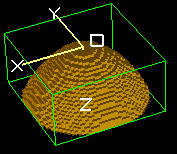It’s convenient to wrap these in a named function for reuse:

```local function paraboloid(x0,y0,z0, a, b)
shape(function(x,y,z) return ((x-x0)/a)^2 + ((y-y0)/b)^2 > (z-z0) end)
end
e(1) { paraboloid(0,0,0, 1, 1) }
```

Other examples:

```pa_define(101,101,1, p, surface=fractional)

local function yplane(y0)
shape(function(x,y,z) return y==y0 end)
end
local function rbox(x0,y0, r)
shape(function(x,y,z)
return (x-x0)^4 + (y-y0)^4 <= r^3
end)
end

e(1) {
yplane(50)
}
e(0) {
rbox(50,50, 40)
notin { circle(50,50, 10) }
}
```

### intersect¶

`intersect`()
```intersect { ... }
```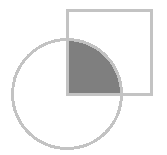This is now a synonym of `within` but provided for improved readability. `intersect` (or `within`) defines a volume that is inside the all the listed shapes (i.e. their “intersected” or boolean “and” volume).

Example:

```e(1) {
intersect {
circle(0,0, 10)
polyline(0,0, 20,0, 20,5)
}
notin { circle(0,0, 5) }
}
```

### revolve_xy¶

`revolve_xy`()
```revolve_xy(angle) { ... }
```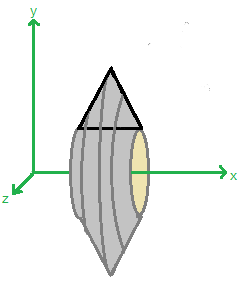Forms a volume of revolution with the given angle (defaults to 360 degrees) of the XY plane around the X-axis (CCW facing down positive X axis). This is similar to `rotate_fill` but can be used in shape context. The contents inside the brackets can be within’s and notin’s.

Example:

```pa_define(21,21,21, p, surface=fractional)
e(1) {
fill {
within { revolve_xy() { within { box(0,1, 7,8) } } }
notin { points(5,5) }
}}
```

The above is the same as this:

```pa_define(21,21,21, p, surface=fractional)
e(1) {
rotate_fill(360) { within { box(0,1, 7,8) } }
}
n(0) {
fill { within { points(5,5) } }
}
```

Furthermore, `within` can be omitted (see omit fill/within commands), so these two are equivalent (standard and simpler syntax):

```revolve_xy() { within { box(0,1, 7,8) } }
revolve_xy() { box(0,1, 7,8) }
```

The `revolve_xy` can be more natural and powerful than `rotate_fill`.

### revolve_yz¶

`revolve_yz`()
```revolve_yz(angle) { ... }
```

This is similar to `revolve_xy` but revolves the YZ plane figure around the Y axis (CCW facing down positive Y axis).

It is equivalent to `rotate_y(90) { rotate_z(90) { revolve_xy(angle) { ... } } }`.

### revolve_zx¶

`revolve_zx`()
```revolve_zx(angle) { ... }
```

This is similar to `revolve_xy` but revolves the ZX plane figure around the Z axis (CCW facing down positive Z axis).

It is equivalent to `rotate_z(-90) { rotate_y(-90) { revolve_xy(angle) { ... } } }`.

### extrude_xy¶

`extrude_xy`()
```extrude_xy(z1, z2) { ... }
```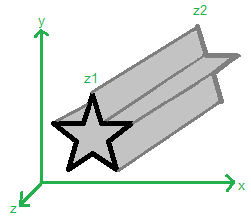Extrudes the figure drawn in the XY plane in the Z direction from Z=z1 to Z=z2.

Example:

```e(1) {
extrude_xy(2, 8) {
polyline(1,1, 10,19, 19,1)
notin { circle(10,8, 2) }
}
}
```

The above is equivalent to:

```e(1) {
within { polyline(1,1, 10,19, 19,1) box3d(-100,-100,2, 100,100,8) }
notin  { circle(10,8, 2) }
}
```

The `extrude_xy` can provide a cleaner approach and can also be intersected with other shapes like `within { extrude() { ... } sphere(...) }`.

If both `z1` and `z2` are omitted, then the extrusion is infinite in the +-Z directions. If only one is omitted, then the omitted one is assumed to be 0. The order of z1 and z2 (swapped) doesn’t matter.

Example:

```e(1) {
extrude_xy() {  ; infinite extrusion in Z
polyline(1,1, 10,19, 19,1)
notin { circle(10,8, 2) }
}
}
```

Note that `e(1) { fill { within { extrude_xy(z2,z2) { fill { within { box() } } } } } }` can be written more simply as `e(1) { extrude_xy(z2,z2) { box() } }`.

### extrude_yz¶

`extrude_yz`()
```extrude_yz(x1, x2) { ... }
```

This is similar to `extrude_xy` but extrudes a YZ plane figure in the X direction.

It is equivalent to `rotate_y(0) { rotate_z(90) { extrude_xy(x1, x2) { ... } } }`.

### extrude_zx¶

`extrude_zx`()
```extrude_zx(y1, y2) { ... }
```

This is similar to `extrude_xy` but extrudes a ZX plane figure in the Y direction.

It is equivalent to `rotate_z(-90) { rotate_y(-90) { extrude_xy(y1, y2) { ... } } }`.

### scale¶

`scale`()
```scale(sx) { ... }

scale(sx, sy, sz) { ... }
```

Scales in the x, y, and z directions by the given factors. If sy or sz is omitted it defaults to sx.

This is identical to:

```locate(0,0,0, sx, 0,0,0, sx,sy,sz) { ... }
```

### rotate_x¶

`rotate_x`()
```rotate_x(anglex) { ... }
```

Rotate counter-clockwise looking down positive x-axis.

This is identical to `locate(,,,,,, anglex)` (rot rotation).

### rotate_y¶

`rotate_y`()
```rotate_y(angley) { ... }
```

Rotate counter-clockwise looking down positive y-axis.

This is identical to `locate(,,,, angley)` (azimuth rotation).

### rotate_z¶

`rotate_z`()
```rotate_z(anglez) { ... }
```

Rotate counter-clockwise looking down positive z-axis.

This is identical to `locate(,,,,, anglez)` (elevation rotation).

### cylinder3d¶

`cylinder3d`()
```cylinder3d(x1,y1,z1, x2,y2,z2, r) { ... }
```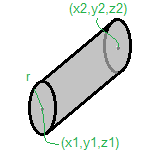Defines a cylinder volume of radius r whose circular bases have centers (x1,y1,z1) and (x2,y2,z2).

This is identical to `locate(x1,y1,z1,1,?,0,?) { cylinder(0,0,?, r,r,?) }` for appropriate values of `?` but is more convenient.

### half_space¶

`half_space`()
```half_space(x1,y1,z1, ux,uy,uz)

half_space(axis, val)
```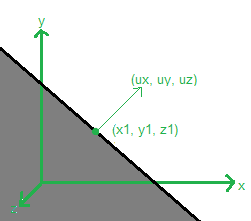Defines the volume below the given plane. The plane is defined by the point (x1,y1,z1) and surface normal vector (ux,uy,uz) on that plane. The fill is in the direction opposite the normal vector. The normal vector length is not important (need not be normalized).

For example, the region x <= 2 is defined by `half_space(2,0,0, 1,0,0)`. The region x + y <= 4 is defined by `half_space(2,2,0, 1,1,0)`.

There is also a short syntax:

```half_space('+x')     -- same as half_space( 0,0,0,  1,0,0)
half_space('-x')     -- same as half_space( 0,0,0, -1,0,0)
half_space('+y')     -- same as half_space( 0,0,0,  0,1,0)
half_space('-y')     -- same as half_space( 0,0,0,  0,-1,0)
half_space('+z')     -- same as half_space( 0,0,0,  0,0,1)
half_space('-z')     -- same as half_space( 0,0,0,  0,0,-1)

half_space('+x', x)  -- same as half_space( x,0,0,  1,0,0)
half_space('-x', x)  -- same as half_space( x,0,0, -1,0,0)
half_space('+y', y)  -- same as half_space( 0,y,0,  0,1,0)
half_space('-y', y)  -- same as half_space( 0,y,0,  0,-1,0)
half_space('+z', z)  -- same as half_space( 0,0,z,  0,0,1)
half_space('-z', z)  -- same as half_space( 0,0,z,  0,0,-1)
```

This is useful for cutting another shape in half, like to define a half-sphere:

```e(1) { intersect { sphere(10,10,10, 5) half_space('+x', 10) } }
```

### e, n¶

`e`()
`n`()
```e(f) { ... }
n(f) { ... }
```

The electrode and non-electrode commands now accept a function as the parameter, which will be queried to determine the potential at the current point. This allows things like defining gradient voltages over electrode surface or analytical potentials in space.

Example:

```; sphere with gradient voltage over surface
pa_define {21,21,21}
local function f(x,y,z)
return x+y
end
e(f) { sphere(10,10,10, 10) }
```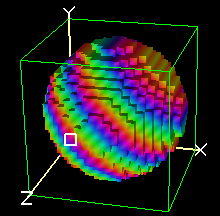Only the coordinate transformations (`locate`) outside the `e` move the potential function. In the following case, the potential at grid points `(x,y,z)` inside the circle will be `f(x-1, y+2, z)`:

```locate(1,-2,0) {
e(f) {
locate(0,2,0) {
sphere(10,10,10, 10)
}
}
}
```

Normally PA’s don’t assign potentials of non-electrode points, allowing Refine to assign their values, but you can use `n` to do this:

```; generate theoretical field plus electrodes.
pa_define {21,21,1}
local function f(x,y,z)  return (x-10)^2 - (y-10)^2 end
local function g1(x,y,z) return f(x,y,z) >  5^2 end
local function g2(x,y,z) return f(x,y,z) < -5^2 end
n(f) { box(-inf,-inf, inf,inf) }  ; assign first (subsequent elecrodes overwrite)
e(f(15,10,0)) { shape(g1) }
e(f(10,15,0)) { shape(g2) }
```

Another example:

```-- circular electrode with linear gradient potential
pa_define {30,30,1, symmetry='planar', mirror='xy'}
local function linear(x1,v1, x2,v2)
return function(x,y,z)
local f = (x - x1) / (x2 - x1)
return (1 - f) * v1 + f * v2
end
end
e(linear(5,100, 10,200)) {
circle(0,0, 20)
}
```
`stl`()

### stl¶

```stl(filename)
stl(filename, x, y, z)
```

Imports an STL file. The STL object can be used like any other shape, including cutting away other GEM objects from it.

If an optional (x,y,z) coordinate (in STL units) is passed, only the fully connected surface closest to that point will be imported. This is one mechanism to assign different parts of an STL different voltages, as an alternative to splitting up the system into multiple STL files. The test of connectivity assumes adjacent triangles touch on at least one vertex.

Example:

```pa_define{30*mm,20*mm,20*mm, 'planar', dx=0.5, surface='fractional'}
locate(0,10,10) {
e(1) {
stl("two_cylinder-1.stl")
}
e(function(x,y,z) return -x/25 end) {
stl("two_cylinder-2.stl")
notin { cylinder3d(25,0,inf, 25,0,-inf, 3) }
}
}
```

Example:

```pa_define{100*mm,20*mm,20*mm, 'planar', dx=1, surface='fractional'}
locate(0,10,10) {
e(1) {
stl("two_cylinder.stl", 5, 15, 0)
}
e(2) {
stl("two_cylinder.stl", 25, 15, 0)
}
}
```

This is implemented in 8.2.0.10. The x,y,z parameters were added in 8.2.0.11.

### inf¶

`inf`
```inf
```

This constant represents infinity. Previously a large number like 1e6 was used.

Example:

```box(-inf,0, inf, 2)
```

## Extensions in 8.1¶

### Macro Support (e.g. variable expansion and preprocessing) - 8.0.4¶

As of SIMION 8.0.4, the GEM processor incorporates a preprocessor that allows things like variable expansion and loops (Issue-I296). which allows GEM files to be more maintainable and easily modified. (This new feature is not described in the 8.0.4 manual except in a footnote.)

A new example GEM file (`examples\geometry\macro.gem`) illustrates the use of this new feature:

```; Example SIMION GEM file using preprocessing to expand Lua variables
; and macros.
; Preprocessing is a new feature SIMION 8.0.4.
;
; Lines starting with '#' or text inside \$() are interpreted as Lua
; code and evaluated.  If \$() contains an expression (rather than a
; statement), the result is outputted.  All other text is outputted
; verbatim.

; These variables are intended to be adjusted by the user.
; Note: both # and \$ syntax are shown for demonstration.
# local ro = 45     -- outer radius, gu
# local ri = 40     -- inner radius, gi
\$(local nshells = 4) ; number of shells

; Now do some calculations on those variables.  Note: math.ceil(x) is
; the ceiling function: it returns the integer closest to but no
; greater than x.
# local hw = math.ceil(ro * 1.1) -- half width, gu
# local nx = hw * 2 + 1          -- num array points in x

; Define array size.
pa_define(\$(nx),\$(nx),\$(nx), planar, non-mirrored)

; Declare a function to be used later.
; This returns the voltage for electrode number n.
\$( local function volts(n)
return n^2 * 10 + n + 1
end )

; This locate centers the shells in the array.
locate(\$(hw),\$(hw),\$(hw), 1, 0,0,0) {
; Now define a loop that creates each shell.
# for n=1,nshells do
#   local rop = ro * n / nshells
#   local rip = ri * n / nshells
electrode(\$(volts(n))) {  ; example calling a function
fill {
within{sphere(0,0,0, \$(rop))}
notin {sphere(0,0,0, \$(rip - 1))}
}
}
# end
}

; Note: the following two lines are equivalent:
fill { within { box3d(0,0,0, \$(nx),0,\$(nx)) }}
# _put("fill { within { box3d(0,0,0, " .. nx .. ",0," .. nx .. ") }}")

; Print output to the log window.  This can be
; useful for debugging.
# print("Hello from GEM file. nx=" .. nx)
```

The example `examples\geometry\polygon_regular.gem` illustrates subroutines in GEM files, allowing you to in effect create your own reusable shapes:

```# -- builds regular polygon in XY plane
# -- with center (x0,y0), radius r, and n sides.
# function regular_polygon(x0,y0, r,n)
polyline(
# for i=1,n do
\$(x0+r*math.cos(theta)), \$(y0+r*math.sin(theta))
# end
)
# end

e(1) { fill {
within { \$(regular_polygon(50,50, 40,6)) }
notin  { \$(regular_polygon(50,50, 20,6)) }
} }
```

When SIMION loads a GEM file containing macros (e.g. `macro.gem`), it processes those macros, writes the result to temporary file (e.g. `macro.processed.gem`), and loads that temporary file.

It also possible to do your own GEM file preprocessing outside of SIMION with your own programming tools. This was more popular prior to the introduction of the above feature but can still be useful in some cases.

Note

This page is abridged from the full SIMION "Supplemental Documentation" (Help file). The following additional sections can be found in the full version of this page accessible via the "Help > Supplemental Documentation" menu in SIMION 8.1.1 or above:
• Helpful Tips for Authoring GEM Files

## surface=auto option in pa_define [8.1.1.25]¶

Obtaining optimal field accuracy of curved surfaces had been tricky prior to the addition of the Electrode Surface Enhancement / Fractional Grid Units feature in SIMION 8.1.1. Electrode surfaces that did not exactly align to PA grid points had to be placed instead on nearby grid points, and certain ways of making this placement were more accurate than others. Cutout volumes (e.g. `notin` GEM commands) were also prone to error (Intersecting within and notin_inside). Consider the example of defining the field between concentric spheres of radii 40 and 80 mm. We can now accurately and simply define this in a GEM file using the (“fractional”) surface enhancement option like this:

```pa_define(101,101,1, cylindrical,xy, surface=fractional)
e(1) { fill { within { circle(0,0, 40) } } }
e(2) { fill { notin  { circle(0,0, 80) } } }
```

However, perhaps there are still cases where you prefer not to use surface enhancement. For whatever the reason (see below), SIMION 8.1.1.25 supports a new `surface=auto` option in the `pa_define` statement of GEM files to achieve a more accurate alignment of surfaces in a simple manner when not using surface enhancement. It can be used simply like this:

```pa_define(101,101,1, cylindrical,xy, surface=auto)
e(1) { fill { within { circle(0,0, 40) } } }
e(2) { fill { notin  { circle(0,0, 80) } } }
```

To understand this feature, let’s consider the way this used to be done in SIMION 7.0/8.0….

You might naively define the concentric spheres like this:

```pa_define(101,101,1, cylindrical,xy)
e(1) { fill { within { circle(0,0, 40) } } }
e(2) { fill { notin  { circle(0,0, 80) } } }
```

However, without care, surfaces could be off effectively by about 0.5-1.0 grid unit (gu), thereby distorting fields by the same amount as if the electrodes were misaligned by this distance in the real physical system (e.g. machining or assembly error). The above is nearly equivalent to this:

```pa_define(101,101,1, cylindrical,xy)
e(1) { fill { within_inside_or_on { circle(0,0, 40.5) } } }
e(2) { fill { notin_inside_or_on  { circle(0,0, 80.5) } } }
```

The `within` and `notin` commands apply a +0.5 grid unit (gu) adjustment to the radius of the fill, which improves field accuracy for the `within`, but for the `notin` we actually want more like a -0.5 gu adjustment. In fact, electrode #2 in the above two examples is visibily off slightly when measured in the View screen. We have typically recommended using `notin_inside` rather than `notin`:

```pa_define(101,101,1, cylindrical,xy)
e(1) { fill { within        { circle(0,0, 40) } } }
e(2) { fill { notin_inside  { circle(0,0, 80) } } }
```

Visually that looks about right if you examine the PA on the View screen, and the calculated field is reasonably good. However, the `notin_inside` (as with `within_inside`) actually applies a nearly 0 gu not -0.5 gu adjustment, so fields are slightly biased. But even this is not ideal in the case of spheres. In fact, the optimal adjustment is often not 0.5 gu but about 0.35 gu, as has been observed for spherical capacitor studies ref and other internal studies on flat and curved shapes rasterized to PA’s.

In SIMION 8.1.1.25, a new `surface=auto` option has been added that automatically applies the best adjustment to `within` and `notin` fill types. It can be used simply like this:

```pa_define(101,101,1, cylindrical,xy, surface=auto)
e(1) { fill { within { circle(0,0, 40) } } }
e(2) { fill { notin  { circle(0,0, 80) } } }
```

and it’s nearly identical to this:

```pa_define(101,101,1, cylindrical,xy)
e(1) { fill { within_inside { circle(0,0, 40.35) } } }
e(2) { fill { notin_inside  { circle(0,0, 79.65) } } }
```

`surface=auto` also affects other shape types like `box`, `parabola`, `hyperbola`, and `polyline` by applying approximately 0.35 gu offsets in the appropriate directions.

`surface=auto` is even useful when electrodes do exactly align to PA grid points (like surfaces that are flat, orthogonal to the axes, and have coordinates that are integral multiples of grid units) because it will automatically get the surfaces boundary points right. Consider defining a 4x4 mm box with a 2x2 mm hole inside, which obviously easily aligns to points on a 1 mm/gu grid. You might naively try to do it like this:

```pa_define(11,11,1, planar,n)
e(1) { fill {
within { box(1,1,  5,5) }
notin  { box(2,2,  4,4) }
} }
```

However, the `notin { box(2,2, 4,4) }` (as is also true when using `notin_inside_or_on`) excludes from the cut the PA grid points on both the inside and border of the 2x2 box. You want to instead use a `notin_inside` to only exclude the grid points on the “inside” (not border) of the 2x2 box:

```pa_define(11,11,1, planar,n)
e(1) { fill {
within       { box(1,1,  5,5) }
notin_inside { box(2,2,  4,4) }
} }
```

That’s pretty simple once you know about it, but it’s easier to do this right just by enabling `surface=auto` like this:

```pa_define(11,11,1, planar,n, surface=auto)
e(1) { fill {
within { box(1,1,  5,5) }
notin  { box(2,2,  4,4) }
} }
```

or even (if you prefer) expressing it like this:

```pa_define(11,11,1, planar,n, surface=auto)
e(1) { fill { within { box(1,1,  5,5) } } }
n(0) { fill { within { box(2,2,  4,4) } } }
```

When `surface=auto` is enabled (and this is also true of `surface=fractional` as of 8.1.1.25), any PA grid points that precisely align to an edge of a `within` or `notin` fill volume are made to be electrode points, which is usually what you want. In other words, a `notin` inside an `e` or a `within` inside an `n` (both of which remove electrode material) will be treated as an “inside” fill to avoid removing the electrode points from the border. This largely avoids fiddling with `within_inside`/`within_inside_or_on`/`notin_inside`/`notin_inside_or_on` fill variants since `within` and `notin` will now just do the right thing. In fact, with `surface=auto`, it’s not usually needed nor recommended to use fill types other than `within` and `notin`; for example, the following is not correct but behaves similar to the “naive” approach mentioned earlier:

```pa_define(11,11,1, planar,n, surface=auto)
e(1) { fill { within{box(1,1, 5,5)}  notin_inside_or_on{box(2,2, 4,4)} } }
```

In fact, the following four GEM files are all correct and generate exactly the same PA:

```pa_define(11,11,1, planar,n)
e(1) { fill { within{box(1,1, 5,5)}  notin_inside{box(2,2, 4,4)} } }

pa_define(11,11,1, planar,n, surface=auto)
e(1) { fill { within{box(1,1, 5,5)}  notin{box(2,2, 4,4)} } }

pa_define(11,11,1, planar,n, surface=fractional)
e(1) { fill { within{box(1,1, 5,5)}  notin{box(2,2, 4,4)} } }

pa_define(11,11,1, planar,n, surface=auto)
e(1) { fill { within_inside_or_on{box(1,1, 5,5)}
notin_inside{box(2,2, 4,4)} } }
```

Please note, however, that although `surface=auto` is generally preferred over `surface=none` (i.e. the default old behavior in SIMION 7.0/8.0) for ease and accuracy reasons, `surface=fractional` (see Electrode Surface Enhancement / Fractional Grid Units) is still preferred over both of these. So, there’s not really a reason to use `surface=auto` unless for some reason you don’t want to use surface enhancement. It’s not really clear what that reason would be except maybe to generate PA’s that are refinable under SIMION 8.0 or to compare accuracy differences with/without surface enhancement. A GEM file using `surface=fractional` can be quickly switched to `surface=auto` without any changes, but switching to `surface=none` may cause 1 gu errors unless additional changes are made to `within/notin` fill types due to the semantic difference these have under `surface=none`. `surface=auto` is somewhat of an anachronism that behaves between `surface=none` and `surface=fractional` but happened to be implemented after both for completeness.

## Changes¶

• 8.2EA-20170324: Major enhancements. See GEM Extensions in 8.2.
• 8.1.3.1: Some changes consistent with 8.2EA-20170324.
• 8.1.2.4: Macro lines beginning with ‘#’ can now be indented with tabs and spaces. This allows cleaner indenting of GEM code.
• 8.1.1.25: A GEM: New `surface=auto` parameter in `pa_define`. This provides a simple way to more accurately position curved surfaces and cutout (`notin`) volumes when not using surface enhancement (`surface=fractional`). This particularly applies to `circle`/`sphere`/`cylinder`, `hyperbola` (Issue-I371), `parabola`, `polyline`, `notin`, and others. Surface enhancement is still better, but if you don’t want to use surface enhancement for some reason, then this is better than nothing. See surface=auto option in pa_define [8.1.1.25].
• 8.1.1.25: C GEM: `within_inside_or_on`/`notin_inside_or_on` now shift the electrode surface outward by a small 0.0001 gu offset. (`within_inside` and `notin_inside` already apply this offset but in the reverse redirection.) This more reliably ensures points “on” the shape boundary are filled even when small numerical round-off occurs in operations like `polyline` and scaling. Example: `fill{within_inside_or_on{box(1,1,5,5)}}` and `fill{within_inside_or_on{polyline(1,1, 5,1, 5,5, 1,5)}}` behave identically now, as expected. [*]
• x GEM/polyline: `polyline` GEM command accuracy has been improved. Previously, the points on the polygon edge might not be optimally filled with the desired electrode/non-electrode point type. This particularly affected `within_inside`/`notin_inside`/`within_inside_or_on`/`notin_inside_or_on` rather than `within`. However, under `surface=fractional`, it also affected `within`/`notin` (but is somewhat cosmetic since electrode point types on the surface are not critical for field accuracy under `surface=fractional`). Example: `polyline(10,5 15,5 15,10 20,10 20,15 15,15 15,20 10,20 10,15 5,15 5,10 10,10)` (cross).
• 8.1.1.25: C GEM/Surface: PA grid points that precisely align to an edge of a `within`/`notin` fill volume are made to be electrode points if `surface=fractional` or `surface=auto` is enabled. In other words, a `notin` inside an `e` or a `within` inside an `n` (both of which remove electrode material) will be treated as an “inside” fill to avoid removing the electrode points from the border. This largely avoids fiddling with `within_inside`/`within_inside_or_on`/`notin_inside`/`notin_inside_or_on` fill variants since `within` and `notin` will now just do the right thing. The default option (`surface=none`) retains the old behavior for compatibility though. Example: `e(1){fill{within{box(1,1,5,5)} notin{box(1,1,5,5)}}}` and `e(1){fill{within{box(1,1,5,5)}}} n(0){fill{notin{box(1,1,5,5)}}}` both create a 4x4 hollow box with infinitesimally thin walls under `surface=auto` or `surface=fractional`. See surface=auto option in pa_define [8.1.1.25].
• 8.1.1.25: c GEM: `polyline`, `points`, and `points3d` commands can now accept an arbitrary number of points. Previously these were limited to 100, 100, and 65 points.
• 8.1.1.25: x GEM: Fix `polyline` problems when using exactly 100 points. [*sf-t1338]